## Extremal combinatorics front page

### Quick description

Extremal combinatorics concerns problems in which one is trying to maximize or minimize a parameter associated with a combinatorial structure, subject to certain constraints. For example, a classic theorem of extremal combinatorics is Turán's theorem, which determines how many edges a graph can have before it is forced to contain the complete graph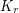as a subgraph. The methods used in extremal combinatorics are extremely varied. This page starts with links to articles about these methods and continues with a short essay about how to decide which methods are appropriate for which problems.

### Prerequisites

A familiarity with basic definitions from graph theory and combinatorics.

### Some important methods in extremal combinatorics

Algebraic methods

The analytic approach: turn sets into functions Quick description ( A technique that is often useful in extremal combinatorics is to replace sets by their characteristic functions. What does this achieve? Well, sometimes it is possible to generalize the statement that one is trying to prove so that it applies not just to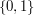-valued functions but to functions with values in larger sets such as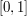,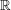or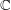. And these generalizations often turn out to be more natural than the original statements and therefore easier to prove. They also allow one to apply a wider range of techniques.)

Compressions

Dimension arguments Quick description ( If you want to find the maximum of a combinatorial quantity, and if the maximum seems to be very far from unique, then try to relate the quantity to the dimension of a certain vector space.)

Direct combinatorial arguments

Fourier techniques

Hilbert space arguments

The probabilistic method Quick description ( If you are trying to optimize a parameter associated with a combinatorial structure, and if the extremal examples appear to be highly "spread about" and unstructured, then you may well do best to consider examples that have been generated randomly. You are unlikely to prove an exact formula this way, but impressively sharp results can be obtained, often of results that nobody knows how to prove in any other way. )

Quasirandomness

Topological methods

### Which of the above methods is the best one to use?

#### Six Examples

Consider the following six problems about collections of sets.

1. For which values of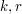andis it possible to find a system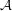of subsets of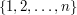such that every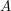inhas sizeand every subset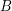ofof size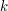is contained in precisely one set in?

2. Let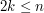and letbe a collection of subsets ofsuch that every set inhas sizeand no two sets inare disjoint? How big canbe?

3. Suppose thatis a collection of subsets ofsuch that every setthat belongs tohas odd size and the intersection of any two different sets inhas even size. How big canbe?

4. Letbe even and suppose thatis a collection of subsets ofsuch that every set inhas size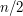and the intersection of any two distinct sets inhas size at most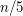. How big canbe?

5. Letbe even and suppose thatis a collection of subsets ofsuch that every set inhas sizeand the intersection of any two distinct sets inhas size at most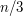. How big canbe?

6. Let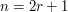, and let the subsets ofof sizebe partitioned intoclasses. How big doesneed to be if no two disjoint sets lie in the same class?

There are strong superficial similarities between all these problems, but the techniques appropriate for solving them are radically different from problem to problem. The first one is closely related to results in algebra, the second can be tackled by clever purely combinatorial arguments (see Example 4 of this argument on double counting for a solution), the third is best rephrased as a problem about vector spaces over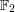(a solution is explained in Example 1 of this article about dimension arguments), the fourth is more fruitfully regarded as a problem about unit vectors in Euclidean spaces (as is explained in Example 1 of this article on the analytic approach to extremal problems), the fifth cannot be solved exactly but estimates can be obtained using tools from probability, and the solution to the sixth is an application of the Borsuk-Ulam theorem from topology.

Incidentally, if you think that the first problem looks rather different from the next four because the next four are about maximizing the size of a set system, consider the following reformulation: let,andbe positive integers with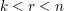. What is the maximum cardinality of a set systemif every set inhas sizeand the intersection of any two distinct sets inhas size at most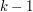? In particular, does such a set system exist with size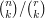? The second question is equivalent to question 1, since if such a system exists, then it has to cover all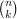sets of sizeexactly once, and each set of sizecovers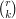sets. And the converse clearly holds too.

Similarly, the last question can be more closely related to the others, though there is a sense in which it is genuinely distinct. One way that one might attempt to prove thathas to be large would be to show that all the classes have to be small, and that would again be a question of maximizing the size of a set system given assumptions about intersections. However, this approach does not give anything like the best bound: it really is a question about partitioning.

### General discussion

If six such similar problems can need such different techniques for their solutions, is there any good way of predicting in advance, when one is faced with a problem in extremal combinatorics, which technique is most likely to work? If one could give a straightforward algorithm for this, then extremal combinatorics would be a duller subject than it is, but there are certainly some useful principles that can help one settle more quickly on a good technique.

A good general piece of advice to start with is to think hard about what you believe the extreme examples should look like. This can make a big difference to how best to tackle the problem. For example, consider the difference between Ramsey's theorem and Turán's theorem. To be continued ...